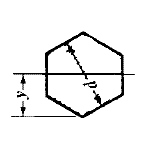Area Moment of Inertia Section Properties: Hexagon Calculator

Area Moment of Inertia Section Properties: Hexagon Calculator

Area Moment of Inertia Section Properties of Hexagon Feature Calculator and Equations. This engineering calculator will determine the section modulus for the given cross-section. This engineering data is often used in the design of structural beams or structural flexural members.

 Description Equation Area Moment of Inertia Section Properties = ISection Modulus = Z = I/yRadius of GyrationA = areay = Distance to neutral axisNote: Units are either inches (left column) or mm (right Column).Membership Register | LoginHomeEngineering Book StoreEngineering ForumExcel App. DownloadsOnline Books & ManualsEngineering NewsEngineering VideosEngineering CalculatorsEngineering ToolboxGD&T Training Geometric Dimensioning TolerancingDFM DFA TrainingTraining Online EngineeringAdvertising CenterCopyright Notice